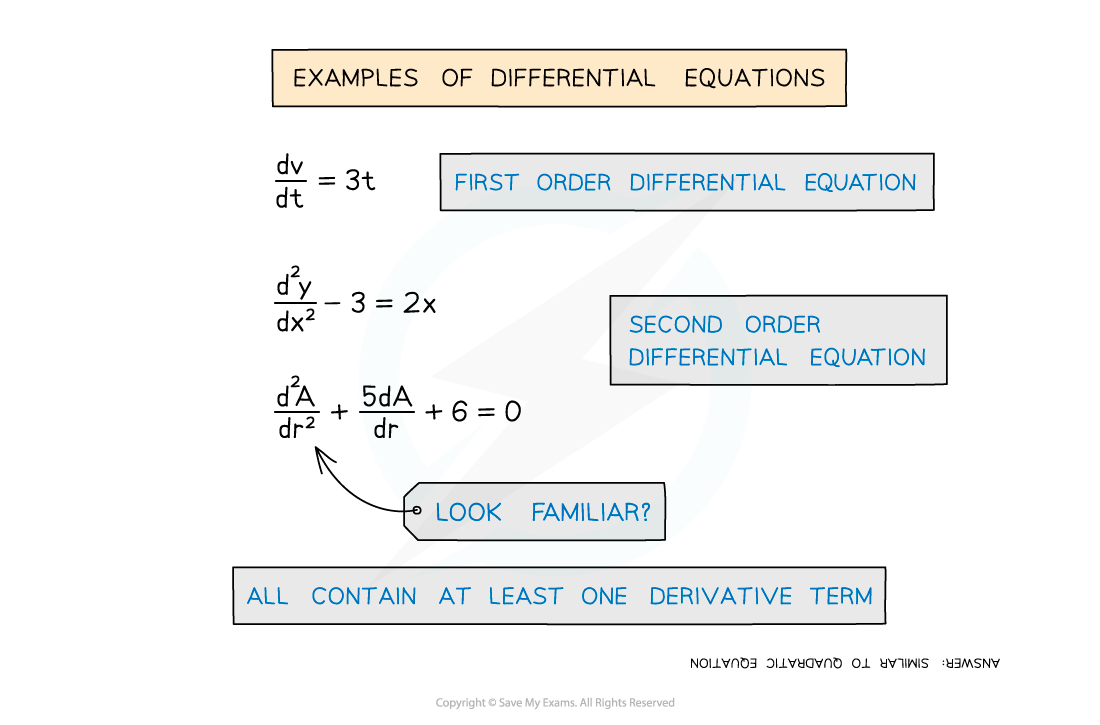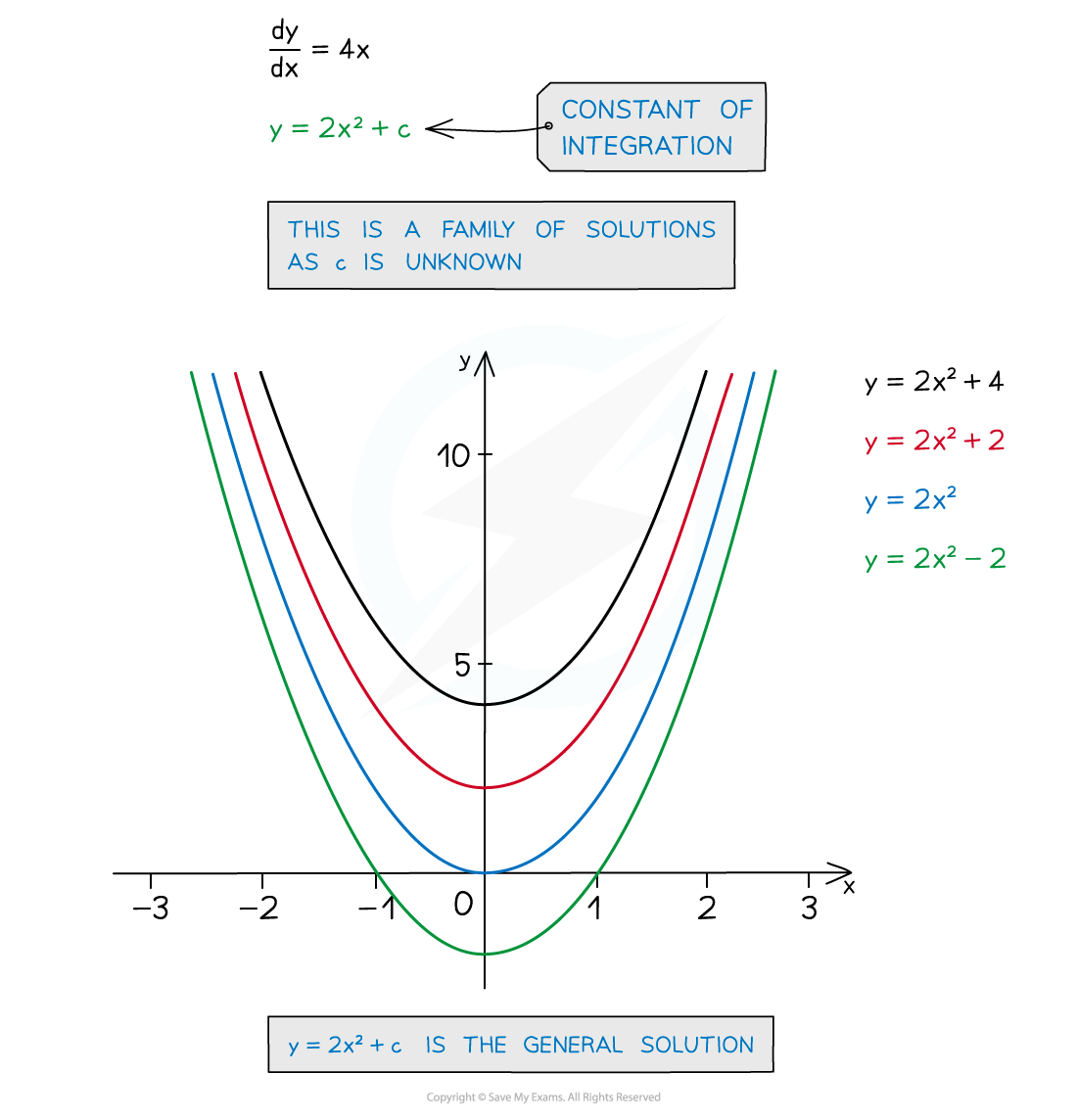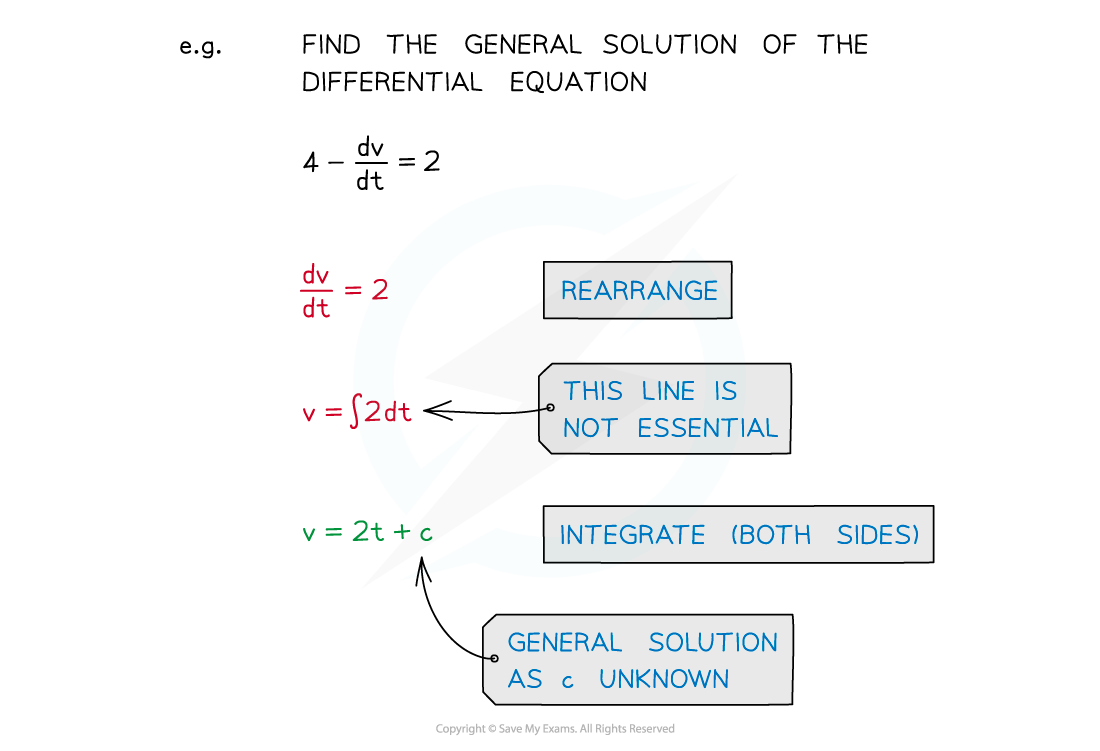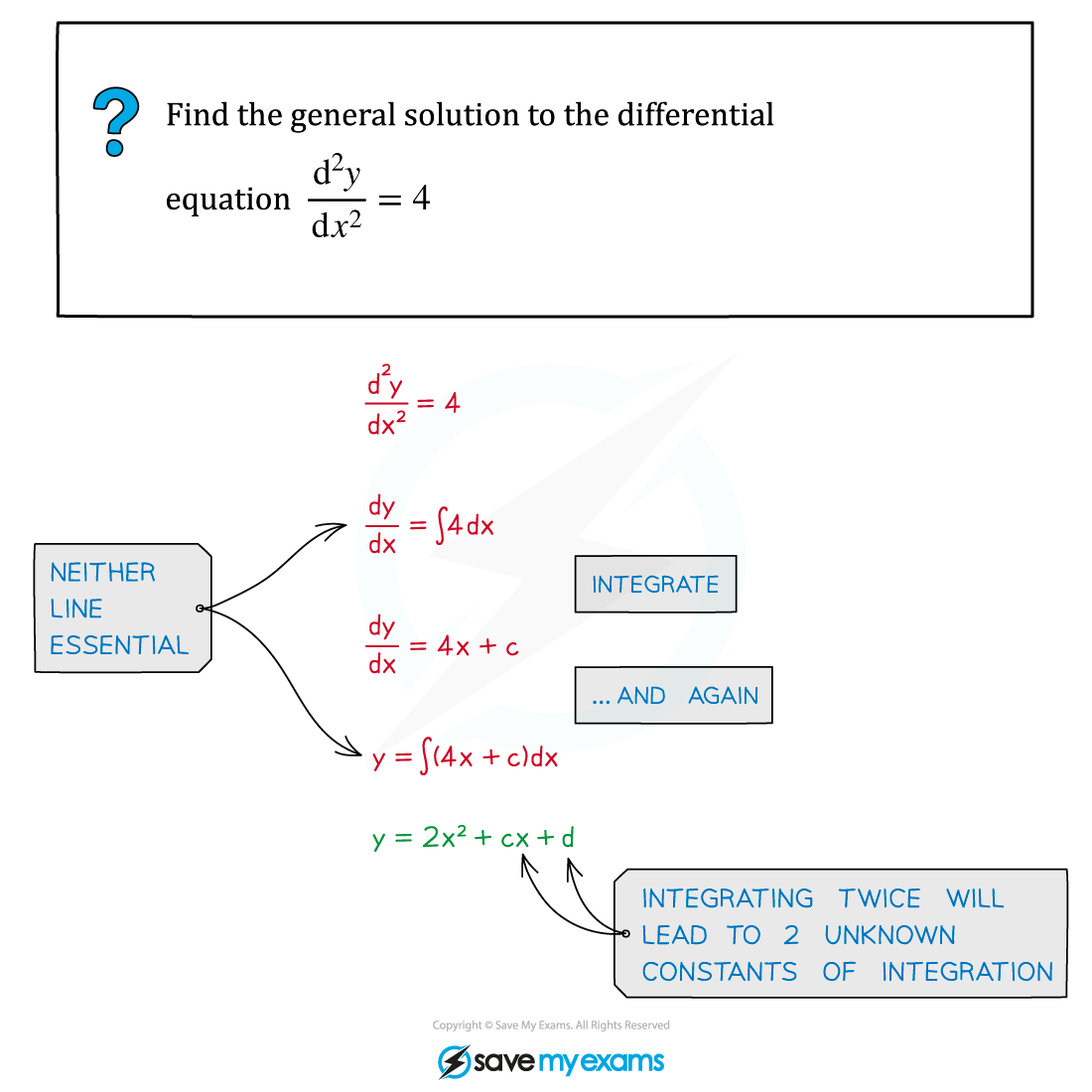# CIE A Level Maths: Pure 3复习笔记5.2.1 General Solutions

### General Solutions

#### What is a differential equation?• Any equation, involving a derivative term, is a differential equation
• Equations involving only first derivative terms are called first order differential equations
• Equations involving second derivative terms are called second order differential equations

#### What is a general solution?• Integration will be involved in solving the differential equation
• ie working back to “y = f(x)
• A constant of integration, c is produced
• This gives an infinite number of solutions to the differential equation, each of the form y = g(x) + c  (ie  y = f(x)  where  f(x) = g(x) + c)
• These are often called a family of solutions …
• … and the solution y= g(x) + c is called the general solution#### Worked Example